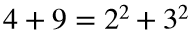# Problem 46701. Compute the nth Naerogahtyp emirp

A Pythagorean prime is a prime number that can be written as the sum of two squares. For example, 13 is a Pythagorean prime because it is. In a similar way, a Naerogahtyp emirp is an emirp that can be written as the sum of two erauqs.
Write a function to find the nth Naerogahtyp emirp.

### Solution Stats

26.19% Correct | 73.81% Incorrect
Last Solution submitted on Apr 25, 2023

### Community Treasure Hunt

Find the treasures in MATLAB Central and discover how the community can help you!

Start Hunting!